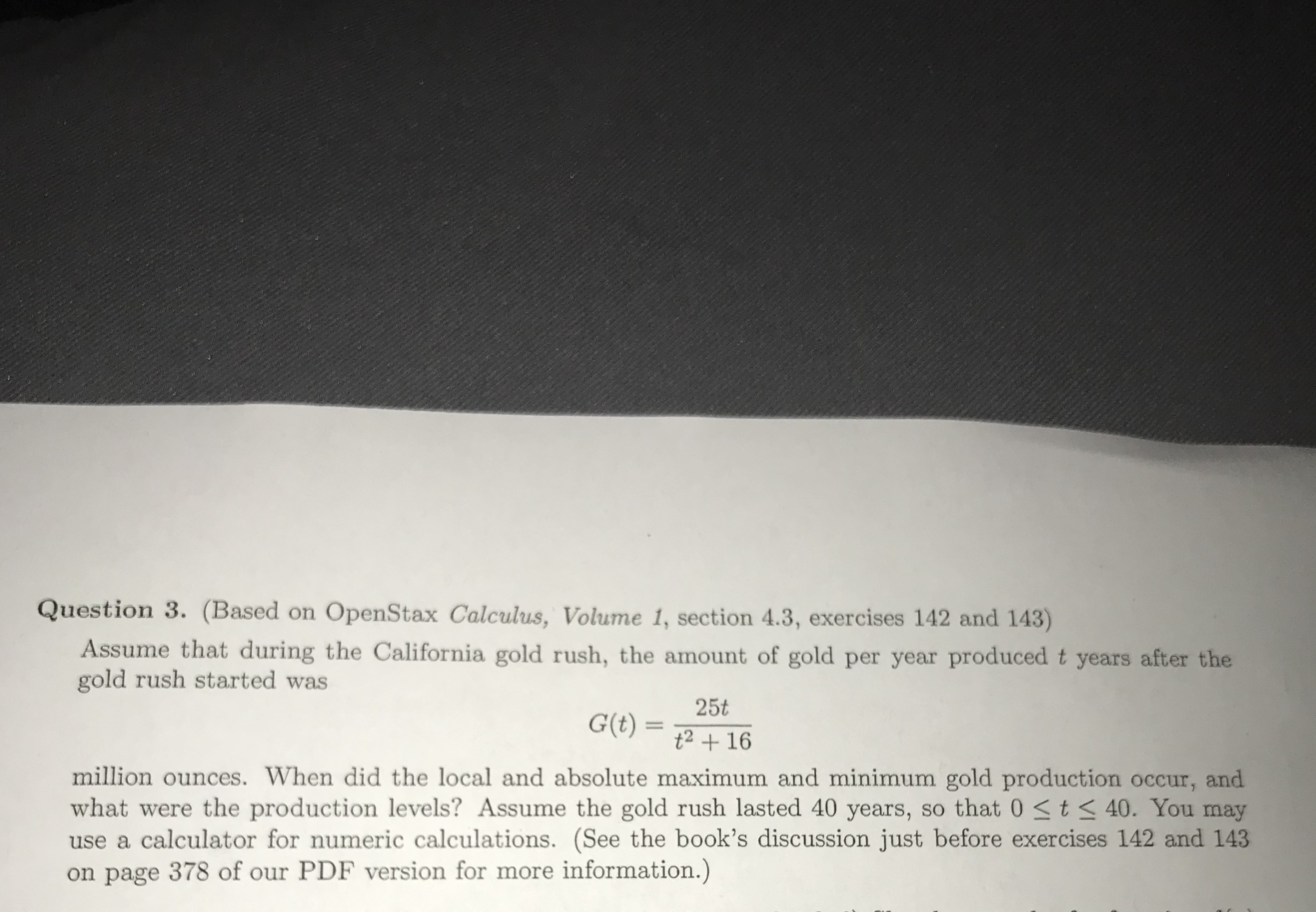Question 3. (Based on OpenStax Calculus, Volume 1, section 4.3, exercises 142 and 143)Assume that during the California gold rush, the amount of gold per year produced t years after thegold rush started was25tt2 +16million ounces. When did the local and absolute maximum and minimum gold production occur, andwhat were the production levels? Assume the gold rush lasted 40 years, so that 0 S t3 40. You mayuse a calculator for numeric calculations. (See the book's discussion just before exercises 142 and 143on page 378 of our PDF version for more information.)

Question

I found the two values of t, but I’m not sure what to do from therehelp_outlineImage TranscriptioncloseQuestion 3. (Based on OpenStax Calculus, Volume 1, section 4.3, exercises 142 and 143) Assume that during the California gold rush, the amount of gold per year produced t years after the gold rush started was 25t t2 +16 million ounces. When did the local and absolute maximum and minimum gold production occur, and what were the production levels? Assume the gold rush lasted 40 years, so that 0 S t3 40. You may use a calculator for numeric calculations. (See the book's discussion just before exercises 142 and 143 on page 378 of our PDF version for more information.) fullscreen
Step 1

We are given equation for G(t)

We can find derivative

Step 2

Now, we can set it to 0

and then we can solve for t

Step 3

Since, time can not be negative

So, we will only consider t=...

Want to see the full answer?

See Solution

Want to see this answer and more?

Our solutions are written by experts, many with advanced degrees, and available 24/7

See Solution
Tagged in

Derivative